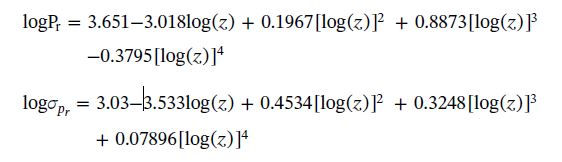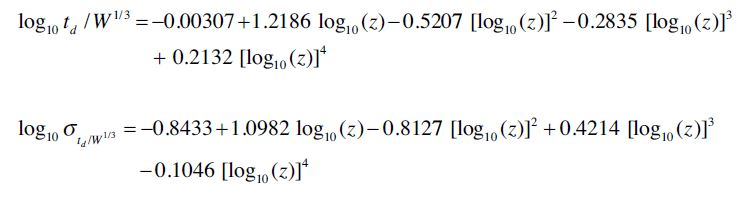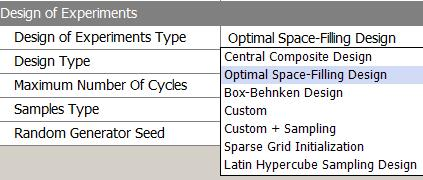## LS Dyna

•arunprabhat12
Subscriber

Hello

I have to do the reliability analysis of a column subjected to blast loading by Monte Carlo technique.

I have modeled column in Explicit Dynamics of the workbench.

How to perform Monte Carlo simulation in the workbench?

•peteroznewman
Subscriber

Please reply with a list of parameters you wish to vary and the range for each parameter.

Please define failure for your reliability definition.

•arunprabhat12
Subscriber

For steel column, 4 random parameters are-

1. Yield strength Fy

2. Modulus of elasticity Es

3. Blast Peak reflective pressure Pr

4. positive blast load duration td

All are normally distributed.

Mean of fy = 240 MPa, cov = 0.06

Mean of Es = 210 GPa, cov = 0.04

The mean and standard deviation of Pr and td are the functions of charge weight (W) and standoff distance (R).scaled distance Z = R / W ^(1/3)

Failure criteria is defined as, Damage index (D) = 1- (P..residual / P..initial)

where, P..initial = Total axial load carrying capacity of column

P..residual = axial load carrying capacity of column after getting damaged by blast load

if D = 0-0.2 : low damage

0.2-0.5: medium damage

0.5-0.8: high damage

0.8- 1 : collapse

MCS procedure:

• Randomly generate variables according to PDF

• Generate the model input file for steel column

• Analyze all finite element models and extract result in terms of axial load carrying capacity (P..residual)

• Calculate damage index

• plot results in terms of CDF of damage index.

•peteroznewman
Subscriber

Hello,

Sorry for the delay in replying.  I typed a reply yesterday, but it disappeared!

Do you know how to add parameters to your model?  Here is an example. That example shows that the Design Exploration toolbox has Response Surface, which creates a DOE from the input parameters. But if you want a Monte Carlo random set of inputs, you can create that in a spreadsheet and copy paste it into the Parameter Set worksheet. Here is a discussion where I generated random values in Excel.

In your example above, you have four parameters. I would recommend using a DOE to study them since each run of the model takes a long time to compute. How long does it take Explicit Dynamics to solve once?  Rather than leaving to random chance the distribution of test points, you can select a DOE that explores that four-dimensional design space in an organized manner. There are a range of experimental types to choose from.It may be very difficult to automatically determine P.residual. That may require manual evaluation of the data.

Regards, Peter

•arunprabhat12
Subscriber

You are right in explicit dynamics this analysis is taking too much time. Even in the coarser mesh of around 100 mm it is taking more than 3 hours. So definitely in Monte Carlo where there will be high numbers of random values, it will take too much time to analyse.

You are right it is difficult to automatically get the values of collapse load, but in MCS we can't do it manually. Is there no way to do this?

I have one more doubt that how the equations of Pr and td (random variables mentioned above) can be entered in Ansys mechanical to define blast loading.

•peteroznewman
Subscriber

I don't know of a way to automatically determine the collapse load.

Your input variables are really W and R and you calculate Pr and td to use inside ANSYS as inputs. I recommend you calculate these outside of ANSYS from the W and R inputs using Excel or mathcad.

•arunprabhat12
Subscriber

Thanks, Peteroznewman for your help.

I can calculate Pr and td outside in Excel.

Because I can't take Pr and td as parameters in Ansys, do I need to create DOE outside to Ansys?

Should I analyze separately the problem for each set of variables in Ansys and manually get the value of collapse load?